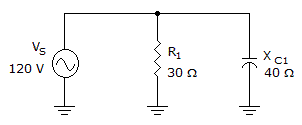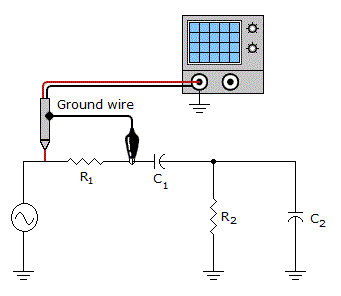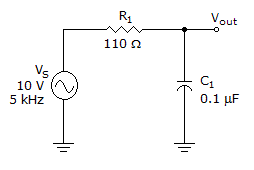# Electronics - RC Circuits

### Exercise :: RC Circuits - Filling the Blanks

1.The phase angle in the given circuit equals___.

 A. 90° B. 36.87° C. 0° D. 53.13°

Explanation:

No answer description available for this question. Let us discuss.

2.Connecting the oscilloscope as shown in the given figure will _____.

 A. measure the voltage across the source B. measure the voltage across C1, R2, and C2 C. short R1 D. short C1, R2, and C2

Explanation:

No answer description available for this question. Let us discuss.

3.If the given circuit is used as a filter, the cutoff frequency is _____.

 A. 5 kHz B. 14.47 kHz C. less than 5 kHz D. more than 20 kHz

Explanation:

No answer description available for this question. Let us discuss.

4.

In a series RC circuit, the phase difference between the capacitor and the resistor voltages ___.

 A. depends on the values of R and C B. equals 0° C. equals 90° D. depends on the value of the input voltage

Explanation:

No answer description available for this question. Let us discuss.

5.

If the impedance equals 2 kin a parallel RC circuit, the admittance equals ___.

 A. More information is needed in order to find the admittance. B. 2 kS C. 4 kS D. 500 µS